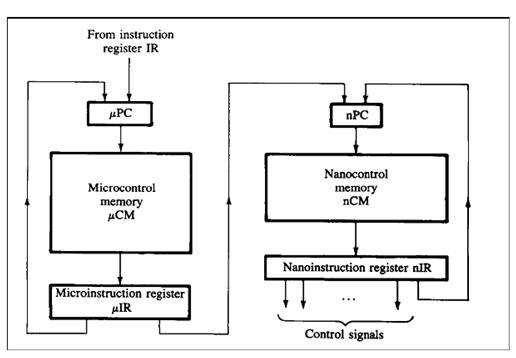9,510 views

A micro instruction is to be designed to specify:

1. none or one of the three micro operations of one kind and
2. none or upto six micro operations of another kind

The minimum number of bits in the micro-instruction is:

1. $9$
2. $5$
3. $8$
4. None of the above

"none or one of the three micro operations of one kind"

it would be "none or one of the 4 micro operations of one kind"

then answer would be two bits or three bits  in vertical microprogramming

and what would be answer in horizontal microprogramming ?

Can't we use concept of nano programming in it? As there are two different kinds of instructions, so we only need to differentiate between two kinds of instruction, which can be done with 1 bit, and max of 4 at a time. So we can implement the control word with 5 bits.

To be clear nano-programming incorporates both vertical and horizontal concepts. First the bits are given to vertical PC then  to vertical decoder and then to horizontal’s PC.Actually the given question incorporates the concept of horizontal μprogramming (also known as decoded form of control signals) and vertical μprogramming (also known as encoded form of control signals)

The $(a)$ part says :

none or one of the three micro operations of one kind

This is referred to encoding form of vertical one since at most one signal can be active in vertical microprogramming since it involves use of external decoder to select one control signal out of the given control signals..

No of bits required for vertical  microprogramming given n number of control signals $=\lceil ( \log_{2} n )\rceil$

Here, $n = 3$

So, no of bits required for part $(a)$ $=\lceil( \log_{2} 3)\rceil= 2$

Now coming to $(b)$ part , it says :

none or upto six micro operations of another kind

at maximum we can have at most $6$ microoperations of another kind at a time. To accommodate that we need decoded form of control signals which is horizontal signals.

So, no of bits required for $(b)$ part

$=$ No of control signals of $(b)$ kind $= 6$

Therefore overall bits required to accommodate both $(a)$ and $(b),$
$= 2 + 6=8-bits$

Besides this, address field, flags etc are also there in a control word. That is why it is asked in the question :

minimum number of bits in the micro-instruction required

Hence, minimum no of bits required  $= 8-bits$

Should it be ceil(log(n+1))  extra 1 for none case?
For this case ceil(log(3+1))
How are we dealing with the "none" case in vertical microprogramming? As a decoder will not have all 0s in it's output for any input.
edited

Sambhrant Maurya

i am having the same doubt!

i think for the none case we will require one more extra bit in both the cases which leads to a total of 9 bits.

EDIT: In (b) for none active case ,we don't need any extra bits it can be done in 6 bits only. so we will only need one more signal in (a) for "none active case". thus total 8 bits.

in the vertical case  , with $2$ bits it can generate  $(00,01,10,11)$ , and none or one of the three can be active here, so for the  none case it is $00$ and for one of the three case it is $(01,01,11)$.

In question it is given that there are two kinds of instruction, let me classify them in two groups G1, G2. In G1 there are 3 microinstructions and in other group G2 there are 6 microinstructions.

in G1: None or one microinstruction is active at a time,  we can implement Vertical programming here as atmost one signal active at a time.

in G2: none or more than one (upto 6) microinstructions active at a time ,  we need Horigontal programming as upto 6 are needed.Therefore toal bits = 2+6 = 8.

(Note that, if we implement Horizontal in G1 also then G1 itself would need 3 bits, and total bits will be 9 (That is option A). But they ask for minimum, therefore we should implement Vertical programming whenever chances.)

Option C is right choice.

Nice explanation thanks, Sachin Mittal!!

@Sachin Mittal 1 sir why are we not considering none case for the horizontal one so if we include then it should ideally be taking 7 bits for horizontal one also right .Please guide me on this

The first condition (None or any one out of 3 operations) gives 4 variations to consider. This uses 2 bits.
The second condition (Any combination of 6 other operations) gives 64 variations to consider. This uses 6 bits.
In total 8 bits.

can u pls exlain a bit more why in the second case 6 bits are [email protected] sarkar
It is because we may need all 6 control signal simultaneously also. That's why for those 6 control signals we need 6 bits in control word.

Any number of signals out of 6 signals can be active at a the point, due to presence of 6 bits and if all are set to 0 then we can say none of them are active.

Both the required conditions are satisfied by using 6 bits for 2nd group of instructions.